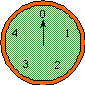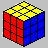Introduction to Abstract Algebraby Henri Picciotto

I have taught these lessons in one form or another to students in Kindergarten through 12th grade, and to teachers, since 1971. Most recently, they have played a crucial role in Space, an advanced high school geometry elective I taught at the Urban School of San Francisco.

Taken together, these lessons are a good introduction to the power and beauty of mathematical structure. The approach is playful and founded on student experience and reflection. The key concept is that of a group, with a special emphasis on the identity and inverse elements, which are essential understandings throughout K-12 mathematics.

Download the following PDF files, which contain a slightly edited version of these lessons, as published in 1994 in Algebra: Themes, Tools, Concepts (ATTC), the textbook I co-authored with Anita Wah.
Lessons
Teacher Notes
The much increased emphasis on geometric transformations in the Common Core State Standards should result in additional relevance for these lessons at the pre-college level.

A closely related topic, grades K-12, is symmetry, as you can see especially in Lessons 4 and 5 of this packet. Find more materials on my Symmetry home page.

The following worksheets for math teachers take the ideas of the above lessons one step further by introducing the concept of isomorphism and the definition of a field. This leads to the construction of the integers and rational numbers. These activities introduce teachers to a generalization and formalization of the arithmetic and algebra they teach. Moreover, they offer a glimpse at the part of university mathematics that is most relevant to teachers' practice.

Abstract Algebra, cont'd (sequel to above lessons, for teachers)
Kinds of Numbers (from ATTC)
In my Space course, I have had good success introducing isomorphisms to students, but not fields. "Kinds of Numbers" works well in Algebra 2, as an introduction to complex numbers.Finally, you may be able to find a use for my approach to solving and enjoying Rubik's cube, which I compiled from various sources many years ago. Alas, I don't remember where I got those ideas.Related pages on this site:
Symmetry
Space
Knot Theory
Map Coloring